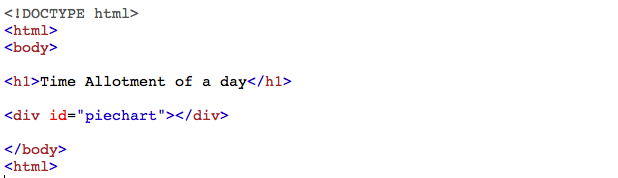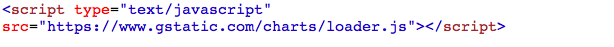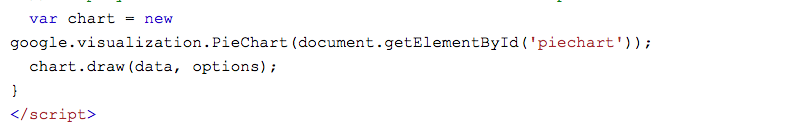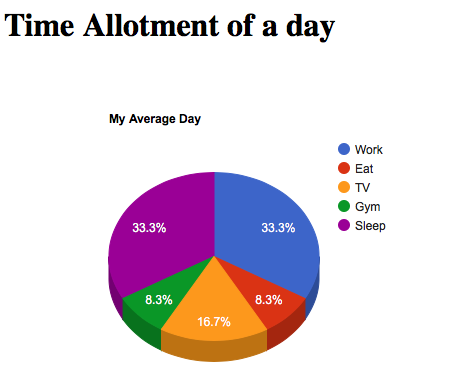Related Articles
• Difficulty Level : Hard
• Last Updated : 15 Nov, 2017

What is a pie chart?
A pie chart (or a circle chart) is a circular statistical graphic which is divided into slices to illustrate numerical proportion. In a pie chart, the arc length of each slice (and consequently its central angle and area), is proportional to the quantity it represents. While it is named for its resemblance to a pie which has been sliced, there are variations on the way it can be presented.

Applications of Pie chart

• Pie charts are generally used to show percentage or proportional data of sales of different items in a business.
• Used in elections to show percentage of votes each party has recieved.
• Used in surveys to depict no of items in each category.
• Broadly used in database and excel sheets.

Add a “div” element with the id “piechart”:Step 2 : Adding a referenceStep 3 : Add a JavaScript functionExplanation : The below code is used to load Google charts :The below code is used to draw a chart and set the user specific values for each division :The code below is used to display the chart inside the “div” element with id=”piechart” :INPUT :

 ```<``html` `lang``=``"en-US"``>``<``body``>`` ` `<``h1``>Time Allotment of a day`` ` `<``div` `id``=``"piechart"``>`` ` `<``script` `type``=``"text/javascript"`` ``src``=``"https://www.gstatic.com/charts/loader.js"``>`` ` `<``script` `type``=``"text/javascript"``>``google.charts.load('current', {'packages':['corechart']});``google.charts.setOnLoadCallback(drawChart);`` ` `function drawChart() {``  ``var data = google.visualization.arrayToDataTable([``  ``['Task', 'Hours per Day'],``  ``['Work', 8],``  ``['Eat', 2],``  ``['TV', 4],``  ``['Gym', 2],``  ``['Sleep', 8]``]);``  ``var options = {'title':'My Average Day', 'width':550, 'height':400};``  ``var chart =`` ``new google.visualization.PieChart(document.getElementById('piechart'));``  ``chart.draw(data, options);``}```` ` ````

OUTPUT :To get a 3d pie chart, set “is3d” option to true :INPUT :

 ```<``html` `lang``=``"en-US"``>``<``body``>`` ` `<``h1``>Time Allotment of a day`` ` `<``div` `id``=``"piechart"``>`` ` `<``script` `type``=``"text/javascript"` `src``=``"https://www.gstatic.com/charts/loader.js"``>`` ` `<``script` `type``=``"text/javascript"``>``google.charts.load('current', {'packages':['corechart']});``google.charts.setOnLoadCallback(drawChart);`` ` `function drawChart() {``  ``var data = google.visualization.arrayToDataTable([``  ``['Task', 'Hours per Day'],``  ``['Work', 8],``  ``['Eat', 2],``  ``['TV', 4],``  ``['Gym', 2],``  ``['Sleep', 8]``]);`` ` `  ``var options = ``   ``{'title':'My Average Day',  'width':550,'height':400,is3D: true};``   ` `  ``var chart = ``  ``new google.visualization.PieChart(document.getElementById('piechart'));``  ``chart.draw(data, options);``}```` ` ````

OUTPUT :My Personal Notes arrow_drop_up